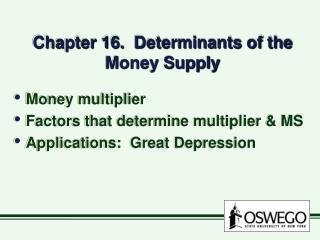Download PresentationChapter 16. Determinants of the Money Supply

Loading in 2 Seconds...

# Chapter 16. Determinants of the Money Supply - PowerPoint PPT Presentation

Chapter 16. Determinants of the Money Supply. Money multiplier Factors that determine multiplier & MS Applications: Great Depression. given problems with simple money multiplier, construct better multiplier cash holdings excess reserves holdings based on M1 = C + D. I. money multiplier.I am the owner, or an agent authorized to act on behalf of the owner, of the copyrighted work described.
Download Presentation## Chapter 16. Determinants of the Money Supply

An Image/Link below is provided (as is) to download presentation

Download Policy: Content on the Website is provided to you AS IS for your information and personal use and may not be sold / licensed / shared on other websites without getting consent from its author.While downloading, if for some reason you are not able to download a presentation, the publisher may have deleted the file from their server.

- - - - - - - - - - - - - - - - - - - - - - - - - - E N D - - - - - - - - - - - - - - - - - - - - - - - - - -
Presentation Transcript
1. Chapter 16. Determinants of the Money Supply • Money multiplier • Factors that determine multiplier & MS • Applications: Great Depression

2. given problems with simple money multiplier, • construct better multiplier • cash holdings • excess reserves holdings • based on M1 = C + D

3. I. money multiplier • how \$1 change in MB will affect MS: M = m x MB

4. 1 + c m = rD + e + c rD = required reserve ratio c = ratio of currency to deposits e = ratio of excess reserves to deposits

5. 1 + .5 example rD = .10 C = \$400 billion D = \$800 billion ER = \$.8 billion or \$800 million m = = 2.5 .10 + .001 + .5 \$1 increase in MB, \$2.5 increase in M

6. II. Factors affecting m & MS • changes in rD • changes in c • changes in e • changes in MB

7. changes in rD • higher reserve requirement • fewer excess reserves to lend • smaller amount of deposit creation smaller multiplier higher rD

8. 1 + .5 example • rD was .10 • suppose it rises to .20 m = .20 + .001 + .5 m = 2.14

9. changes in c • higher c • currency does not expand like deposits • smaller amount of deposit creation smaller multiplier higher c

10. 1 + .8 example • original example: c = .5 • suppose c = .8 m = = 2.00 .10 + .001 + .8

11. changes in ER/D • higher e • banks hold more ER, lend less • smaller amount of deposit creation smaller multiplier higher e

12. 1 + .5 example • original example: e = .001 • suppose e = .005 m = = 2.48 .10 + .005 + .5

13. what affects e? • as interest rates rise • opportunity cost of holding ER rise • (money could be lent out) ER fall higher i

14. expected deposit outflows • must hold more ER

15. Factors affecting MB • MB = MBn + DL • MBn is nonborrowed MB -- open market purchase will increase MBn -- open market sale will decrease MBn • increase MBn will increase M

16. DL is discount loans -- increase as banks borrow from the Fed -- increase as spread between market interest rate and discount rate increases

17. Great Depression 1930-33 • big contraction in M1 • big increases in c, e • depositors withdrew cash • banks increase ER due to increase in deposit outflow

18. as c and e rise, • money multiplier declines • M1 declines by 25% from 1930-33